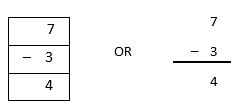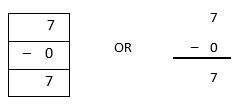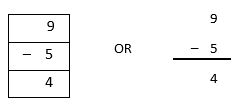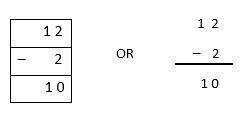## LetsPlayMaths.com

WELCOME TO THE WORLD OF MATHEMATICS

# Class I Subtraction

Basic Subtraction

Subtracting Zero

Subtracting a Number from Itself

Story Subtraction

Subtraction Test

Subtraction Worksheet

## Basic Subtraction

When we remove something from a group of things then the process is known as subtraction or deduction. We use minus(-) sign for subtraction.
There are 5 stars as shown.If we removefrom the group of stars, thenwill remain.
We write it as 5 − 2 = 3
We read it as 5 minus 2 is equal to 3

Examples 1. Subtract 4 from 9.

Solution.Answer can be written as shown below.Examples 2.Subtract 3 from 7.

Solution.Answer can be written as shown below.## Subtracting Zero

When you subtract zero from any number, then the answer would be same number.## Subtracting a Number from Itself

When we subtract a number from itself, the answer will always be zero.## Story Subtraction

In our day to day life we will come across these simple subtractions.

Example 1.There are 9 birds on a tree. If 5 birds fly away, then how many are left on the tree?

Solution.So, 4 birds left on the tree.

Example 2.There are 12 people on a van. If 2 people got down, then how many people remain in the van?

Solution.So, 10 people are there in the van.

## Class-1 Subtraction Test

Subtraction Test - 1

Subtraction Test - 2

Subtraction Test - 3

## Class-1 Subtraction Worksheet

Subtraction Worksheet - 1

Subtraction Worksheet - 2

Subtraction Worksheet - 3

Subtraction Worksheet - 4

Subtraction Worksheet - 5